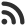Feed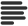Articles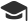Tutorials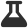Lab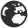CompaniesLeaderboard
 DG Student at BIT Mesra Aug. 30, 2020, 10:30 a.m. ⋅ 811 views

# DXC Placement Aptitude Questions with answers

1. In a poultry farm, 50 hens give 200 eggs in 2 days. In how many days will 20 hens give 400 eggs ?

A. 10
B. 20
C. 15
D. 17

Ans. A

2. If the following numbers are rewritten by interchanging the digits in ten’s place and hundred’s place and then arranging them in the descending order. What will be the second digit of the newly formed fifth number from your right ?
479, 736, 895, 978, 389, 675

A. 3
B. 4
C. 5
D. 6

Ans. C

3. Price of a book increases 15% successively (2 times). What is the new price of the book more compared to that of the old price:

A. 32.25%
B. 23.34%
C. 36%
D. 39%

Ans. A

4. It is known that Meera has 2 children. At least one of them was a girl. Then the probability that 2 of the are girl is :

a)1/2
b)1/4
c)0.36
d)1

Ans. A

5. What is the least number which should be added to 1330 to make it a perfect square?

A. 28
B. 31
C. 39
D. 48

Ans. C

6. One gear of pulley rotates at a speed of 3 revolutions per second, another gear rotates at 5 revolutions per second. If both start together, after how many seconds will they be together again?

A. 12
B. 27
C. 15
D. 38

Ans. C

7. On dividing a number by 342, we get 47 as remainder. If same number is divided by 18, then find the remainder.

A. 18
B. 11
C. 13
D. 15

Ans. B

8. In what ratio must rice at Rs 9.30 per Kg be mixed with rice at Rs 10.80 per Kg so that the mixture be worth Rs 10 per Kg?

A. 6:5
B. 8:7
C. 3:7
D. 6:1

Ans. B

9. The ratio, in which tea costing Rs. 192 per kg is to be mixed with tea costing Rs. 150 per kg so that the mixed tea when sold for Rs. 194.40 per kg, gives a profit of 20%.

A. 1:2
B. 2:5
C. 3:5
D. 3:7

Ans. B

10. A bag contains 4 white, 5 red and 6 blue balls. Three balls are drawn at random from the bag. The probability that all of them are red, is:

A. 1/22
B. 3/22
C. 2/91
D. 2/77

Ans. C

Events

Nov. 28, 2018, 5:30 p.m.

Python from zero to hero

place Delhi

Aug. 13, 2018, 5:30 p.m.

Python from zero to hero

place Bangalore ( HackersFriend office BTM Layout)